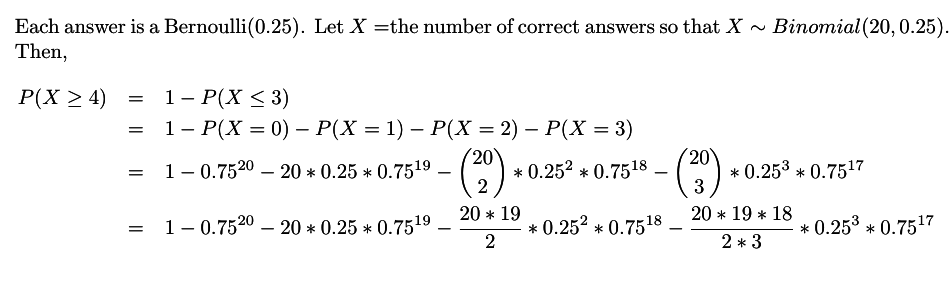Tags
#Exam #has-images
Question
A test consists of 20 multiple-choice questions, each with 4 possible answers. If the student guesses on each question, find the probability that at least 4 questions are correct.Tags
#Exam #has-images
Question
A test consists of 20 multiple-choice questions, each with 4 possible answers. If the student guesses on each question, find the probability that at least 4 questions are correct.

Tags
#Exam #has-images
Question
A test consists of 20 multiple-choice questions, each with 4 possible answers. If the student guesses on each question, find the probability that at least 4 questions are correct.If you want to change selection, open document below and click on "Move attachment"

#### pdf

owner: mmcarli - (no access) - Practice Midterm.pdf, p2

#### Summary

status measured difficulty not learned 37% [default] 0

No repetitions